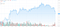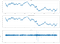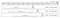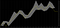# Pragmatic Deep Learning Model for Forex Forecasting

## What is Forex?

`Spread = Buy - Sell = 1.28820 - 1.28816 = 0.00004 = 0.4e-4`
`Spread = 0.4e-4 = 0.4 pips`
`1.28827 - 1.28816 = 0.00011 = 1.1 pips`

## Tick Data

`Date                  | Sell    | Buy20200930 00:00:00.220 | 1.28643 | 1.2865420200930 00:00:00.322 | 1.28643 | 1.2865320200930 00:00:01.025 | 1.28641 | 1.2865520200930 00:00:01.754 | 1.28641 | 1.2865420200930 00:00:03.403 | 1.28642 | 1.2865320200930 00:00:04.204 | 1.28642 | 1.2865520200930 00:00:04.255 | 1.28643 | 1.2865420200930 00:00:04.356 | 1.28644 | 1.2865620200930 00:00:05.520 | 1.28645 | 1.2865720200930 00:00:05.853 | 1.28647 | 1.28657`

## Open High Low Close Data

`Date           | Open    | High    | Low     | Close20200930 00:00 | 1.28643 | 1.28663 | 1.28641 | 1.2865920200930 00:01 | 1.28663 | 1.28675 | 1.28649 | 1.2864920200930 00:02 | 1.28649 | 1.28650 | 1.28627 | 1.2863020200930 00:03 | 1.28630 | 1.28648 | 1.28626 | 1.2863820200930 00:04 | 1.28639 | 1.28647 | 1.28635 | 1.2864020200930 00:05 | 1.28641 | 1.28654 | 1.28641 | 1.2865120200930 00:06 | 1.28650 | 1.28655 | 1.28648 | 1.2865320200930 00:07 | 1.28653 | 1.28654 | 1.28647 | 1.28649`

# 1 — Data Sourcing

`Date,High,Low2010-01-01 00:00,1.61673,1.616592010-01-01 00:01,1.61670,1.61670...2020-10-01 23:58,1.28852,1.288382020-10-01 23:59,1.28853,1.28846`

# 2 — Data Preparation

## Time Interval and OHLC

`df['HLAvg'] = df['High'].add(df['Low']).div(2)`

## SmoothingSMA with 14 periods on GBPUSD 1-minute chart. Chart is from TradeView.com and the data source is FXCM.
`df['MA'] = df['HLAvg'].rolling(window=14).mean()`

## Stationarity

`df['Returns'] = np.log(df['MA']/df['MA'].shift(1))`
`df['MA'] = df['MA'].mul(np.exp(df['Returns'].shift(-1))).shift(1)`Time series with the simple moving average and the log returns

## Train, Test Split

`df = df[df.shape % batch_size:]`
`df_train = df[:- val_size - test_size]df_val = df[- val_size - test_size - window_size:- test_size]df_test = df[- test_size - window_size:]`

## Scaling

`scaler = MinMaxScaler()train_values = scaler.fit_transform(train_df[['Returns']].values)...test_values = scaler.transform(test_df[['Returns']].values)`
`df['Returns_Prediction'] = scaler.inverse_transform(df[['Returns_Prediction_Scaled']].values)`
`joblib.dump(scaler, 'scalers/scaler.bin') # For persisting to file...scaler = joblib.load('scalers/scaler.bin') # For loading from file`

## Window SizeAn illustration of samples shifted by 1 each. Image by author
`batch_size = 32window_size = 8 * batch_size # 256 minutes, 4.3 hours`

## Converting Samples

`def convert_raw_samples_to_model_samples(scd_log_rtns, window_size):    X, y = [], []    len_log_rtns = len(scd_log_rtns)    for i in range(window_size, len_log_rtns):        X.append(values[i-window_size:i])        y.append(values[i])    X, y = np.asarray(X), np.asarray(y)    X = np.reshape(X, (X.shape, X.shape, 1))    return X, y`

# 3 — Model Training

`model = Sequential()model.add(LSTM(76, input_shape=(X.shape, 1), return_sequences = False))model.add(Dropout(0.2))model.add(Dense(1))model.compile(loss="mse", optimizer='Adam')`

# 4 — Predictions

`y_pred = model.predict(X)df['Pred_Scaled'] = np.pad(y_pred.reshape(y_pred.shape), (window_size, 0), mode='constant', constant_values=np.nan)df['Pred_Returns'] = scaler.inverse_transform(df[['Pred_Scaled']].values)df['Pred_MA'] = df['MA'].mul(np.exp(df['Pred_Returns'].shift(-1))).shift(1)`

# Continuing and Expanding the Research

## Sequence-to-Sequence Forecasting

`1.2752, 1.2751, 1.2754, 1.2756 -> 1.2758, 1.2760, 1.2761`

# Part Two: Using the Model from a Trading PlatformBacktesting of profit simulation of £1000 between 24/08/2020 and 30/08/2020 using this model. To be discussed in part 2. Captured from cTrader

# References

## Towards AI

### By Towards AI

Towards AI publishes the best of tech, science, and engineering. Subscribe with us to receive our newsletter right on your inbox. For sponsorship opportunities, please email us at pub@towardsai.net Take a look

Written by

## Towards AI

Written by

#### Software Architect from London with a certificate in Quant Finance and a background in Software Engineering. Passionate about machine learning, C# and Python